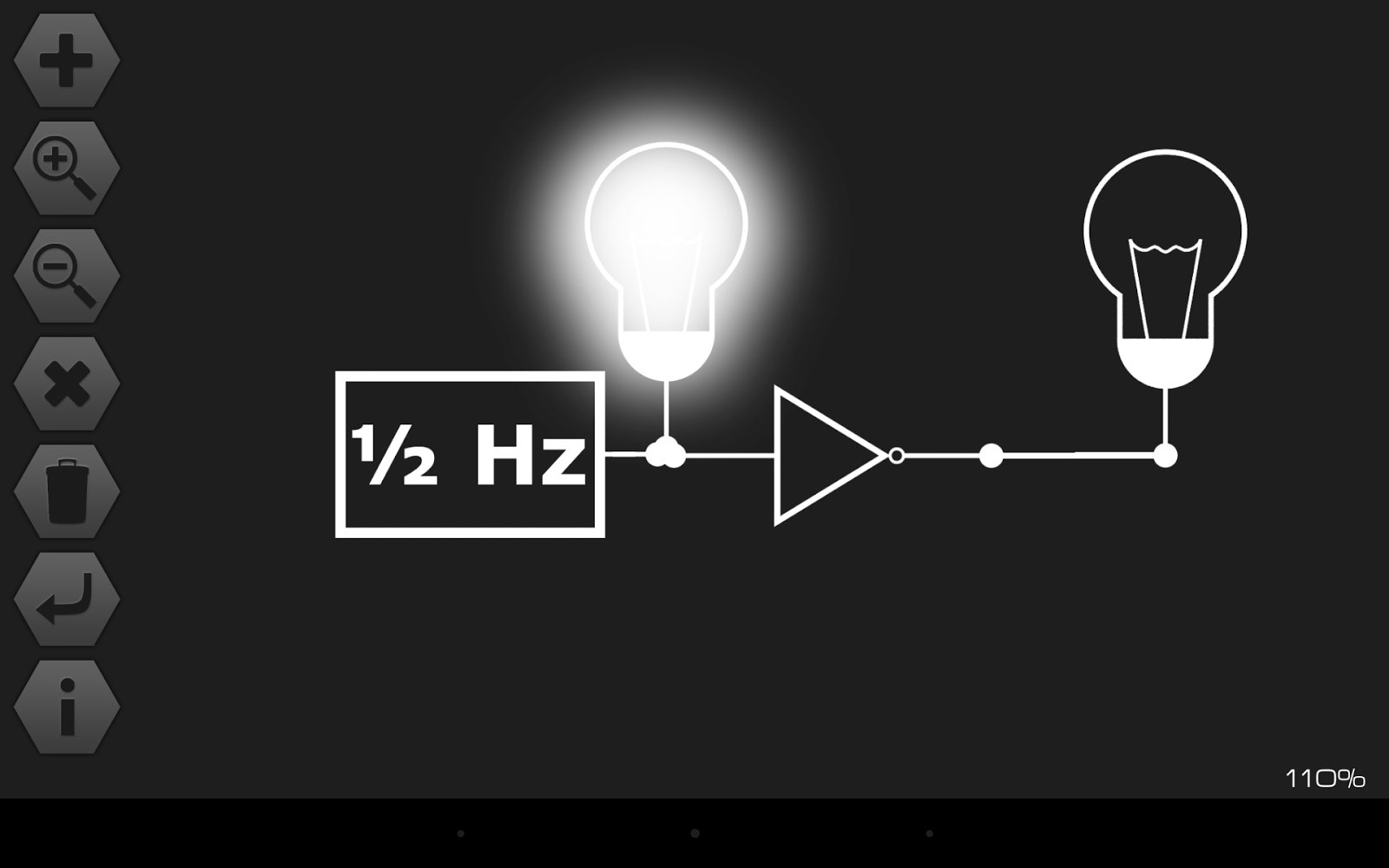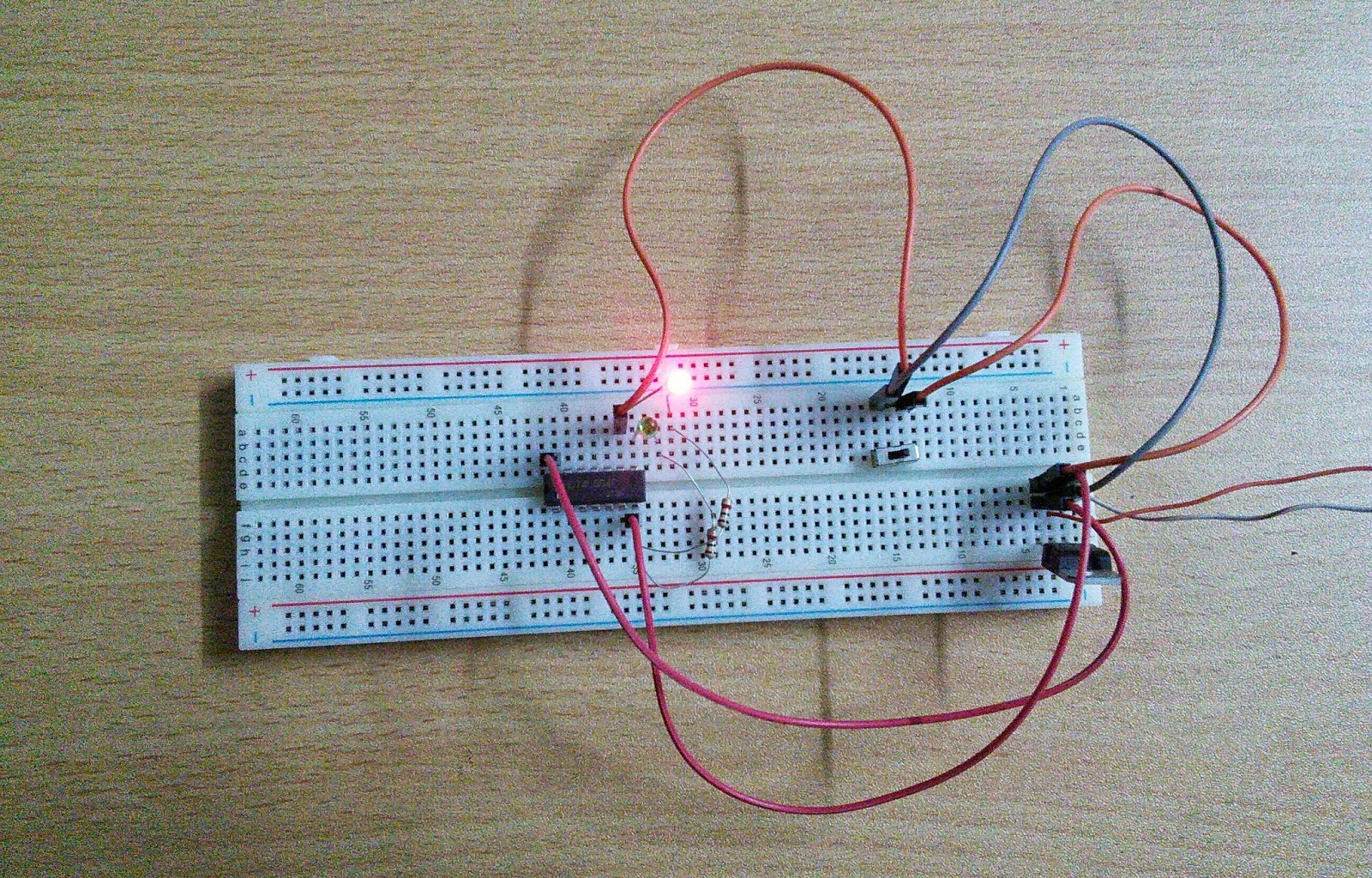## Friday, April 26, 2013

### NOT GATE

Explanation & Construction of NOT Gate

Good evening everyone. Again welcome to my blog. This post is about a logic gate called NOT & I’m using many different method for demonstrating this logic gate.

What is NOT Gate?

### That’s why NOT gate is also called “Inverter” as it inverts the input.

So it is such a gate that will provide a "LOW" output when Input is "HIGH" & provide a "HIGH" output when input is "LOW".

Symbol

Truth Table

Circuit Diagram Using NPN Transistor

First in technical terms, here 2N3055 is a NPN transistor designed for switching applications. Main property of this configuration is that it provides 180 degree phase shift. So if the input is high the output will be low. Biasing is done in such a way that the operating point is near the origin of the transfer characteristics. Thus when a high voltage is given to the base, the transistor enters into the active mode and quickly get into saturation. Thus a current will flow through R1. Due to this the collector voltage goes low as result of the drop across the R1. Now if a low voltage is given to the base, transistor is cut off and no current flows through R1. Thus the collector voltage is almost equal to the input 12V. This is how it works as a not gate.

In easy words, as we all know, transistor can be used for switching purpose and a NPN transistor requires a positive voltage in its Base to turn on. The input is given to the transistor via a 4.7Kohm resistor and a press button, and the output is taken from the collector of the transistor. If the press button is in normal condition there will be no connection between the positive 12V and the Base, so the transistor will stay at OFF. In this case the Input will be zero. but if the Transistor is OFF then no current will pass through the transistor's Collector to Emitter as a result there will be voltage present at the output. So when the input is 0 the output is 1. Assuming that 0 for no voltage or OFF and 1 for some voltage present or ON. Now if the press button is pressed there will be current flowing from the +12V DC through the 4.7Kohm resistor to the Base of the transistor causing it to turn ON. As the transistor is turned on its Collector to Emitter junction resistance drops significantly making it like a short. So all the current will pass through the transistor the the ground. As a result the output voltage will also be zero. So when the input is 1 the output is 0.

More Information:

Almost any NPN transistor will perform the same. So the transistor can be replaced with D400/2N2222. A LED can be connected with the input and the output to get clearer action of a NOT gate using this circuit below.

Let me explain the figure above. We can explain this circuit for two different instances. One if the Press button is pressed or if it isn't pressed.

When press button is not pressed:

As you can see from the construction if the button is not pressed the base and the Green LED won't get any current from the positive terminal. Being a NPN Transistor, it will not turn on as there is no positive voltage on the Base. And the Green LED won't glow as it's not getting any positive voltage either. So the input will be Zero in this case. But if the input is zero and as a result there will be no current flow between the Collector & emitter of the transistor and they can be treated as open. So the Blue LED will start glowing. Current will flow through the 1Kohm resistor connected with the positive terminal through the LED to the ground. So when there is no input we will get an output.

When press button is pressed:

So, if the press button is pressed that means the input now is One or something. And now current can flow from the positive terminal to both the Base of the transistor and the Green LED. So, the Green LED which indicates the input status will glow. As there is positive voltage present at the Base of the transistor it will start to conduct. And a on transistor's Collector and Emitter can be treated as short. That means the Blue LED which represents the output is now shorted out and it won't glow. So when the input is something, the output is zero.

Which is pretty much the NOT Gate.

Not Gate with Simulation software:

Let's test the Gate in a simulation software. First demonstration will be using PSpice Schematics 9.2 on PC running on windows operating system.

From the pictures its clear that when the Input is 0V or Low the output is around 20V which can be considered high.
And when the input is high, 5V the output is around 200mV which can be considered as low!

Second simulation is on a Nexus 7 device running on Android operating system and it is done with a simple application called logic simulator.Input 1 and Output is 0Input 0, Output is 1
What I have done is simply applied a half hertz frequency to the input of a NOT gate and added two light bulbs to see the effect. When the input is high(in the first image) the input light is on and because of NOT operation the output light is off. And in the second image when the input goes low and the light turns off, the output goes high and the output bulb lights up.

Demonstration:

For demonstrating the whole idea of NOT gate we can use very simple circuit with logic IC. Logic ICs are readily available in the market. For this you can get either the TTL 7404 or CMOS 4069. Both of them are inverter and have the same pin configuration. Each of these ICs has 6 inverters inside of them. We will use this IC to demonstrate NOT gate. Let's take a look at the circuit diagram first.

Now lets take a look at the original project and will discuss it later.When Input is 0 or low the output is High or 1When the input is 1 or high the output is low or 0

So the diagram is pretty simple. We will use only one input and one output of the Hex inverter. I have used the pin 8 and 9, you can use any of the input or output pins. Pin 14 and 7 is used for powering up the IC. I have used 5V to run this circuit. 5V is obtained from a regulator IC which is 7805. See how it is done. As I'm not going to draw much current from the source I haven't used any smoothing capacitor. Anyway back to the main thing, The IC is powered up and a SPDT switch ( Single Pole Double Throw) is used for giving 0 and 1 as input. The input is connected to both pin 9 for giving the logic circuit an input and powering up the LED. When the Input is low that means 0V the RED LED attached to it won't glow but as described above NOT gate will give a High output when its input is low. And that's exactly what is seen in the first picture. RED LED off and Green LED is glowing.

Now if we change the position of the SPDT switch ( Zoom in for seeing it, sorry for the low quality image) the Input will go high or 5V the RED LED will start glowing ( Second picture) and at the same time the output will go low and the GREEN LED won't glow.

So, a very simple demonstration of NOT gate can be done by this method.

CMOS CD 4069 Datasheet.
TTL 7404 Datasheet.

Read my other entries, go to the Index.

That's all about NOT gate. Hope it will help.